# Inheritance and types of Inheritance in C++

Inheritance is a one of the most important feature of object oriented programming.

Inheritance allow an object of one class to acquire the properties of another object of another class.

It supports the reusability and support hierarchical classification.

The class which inherits the another class is called derived class and the class which inherited is called base class.

#### Syntax of creating a derived class:

Example:

In the above syntax, class A is a base class, class B is a derived class. Here class B is derived publicly.

Example:

In the above syntax, class A is a base class, class B is a derived class.

Here class B is derived privately.

Example:

If no access specifier is given , by default derivation is private.

Public & Private Inheritance: In a C++ when a class derived and public access specifier is used.

All the public member of base class can be accessed directly in the derived class but when private access specifier is used, then even public member of base class can not access directly in derived class

Public Inheritance: When a public access specifier is used, the public member of the base class are the public member of the derived class.

Example:

Output

Description: In the above program, class B derives class A  means  class A is a base class, class B is a derived class.

During inheriting a class public access specifier is used therefore public member of base class A can directly access in derived class B.

In above program “x” is a public member of base class A but it can directly access in derived class B.

Private Inheritance: When a class derived and private access specifier is used, then an object of derived class has no permission to access even public member of base class directly in main() function.

In such case, the public member of base class can be accessed using public member function of the derived class.

Example : When a class derived and private access specifier is used, then an object of derived class has no permission to access even public member of base class directly in main() function.

Output

Solution of such problem is that the public member of base class can be accessed using public member function of the derived class.

Output

### Derived class can not access private data of base class

In a C++  programming ,derived class can’t access private data of base class.

If we will try to do so we will get an error message.

Example: In the below program we are trying to access the private data of base class in a derived class.

Output

Protected access specifier with public  derivation : when a protected access specifier is used, the protected member of the base class are the protected member of the derived class.

Protected access specifier : A protected access specifier is similar to the private only difference is that it has a access to their derived classes.

Example 1

Output

Example 2

Output

## Types of Inheritance

There are following types of inheritance supported by C++:

1.  Single Inheritance
2.  Multilevel Inheritance
3.  Multiple Inheritance
4.  Hierarchical Inheritance
5. Hybrid Inheritance
6. Multipath Inheritance

Single inheritance: When only one class derived a class and derived class is not used as a base class such type of inheritance are called Single inheritance.

Here, X is a base class, Y is a derived class. In a single inheritance there is only one base class and one derived class.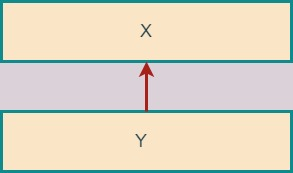Single Inheritance

Multilevel Inheritance When a class derived from another derived class such type of inheritance is called multilevel inheritance.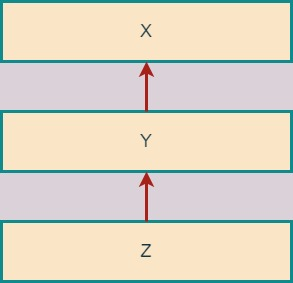Multileve Inheritance

Here X is s base class for Y, Y is a derived class from X and Y is also base class for Z. Z is a derived class from Y.

Multiple Inheritance :  When a single class is derived from two or more then two class then such type of inheritance is called multiple inheritance.

X & Y are the base class for Z , Z is deriver class. Class Z  is derived from Class X & Y.

Hierarchical Inheritance: When a two or more classes are derived from a single base class is  known as hierarchical inheritance.

W is a only base class and X,Y & Z are derived class. X,Y & Z are the derived from W.

Hybrid Inheritance :  The combination of two or more inheritance are called hybrid inheritance.

In this type two types of inheritance are use single inheritance and multilevel inheritance.

Single Inheritance:  W is a base class, Z is a derived class. Single inheritance has only one base class and one derived class.

Multilevel Inheritance:X is s base class for Y, Y is a derived class from X and base class for Z. Z is a derived class from Y.

Multipath Inheritance : When a class derived from two or more classes that are derived from same base class such type of inheritance is known as multipath inheritance.

W is a base class and X & Y are derived class. The class X & Y are the derived  from W.

Further Z is derived from X & Y. means X & Y  is a base class for Z and Z is a derived class.

Here, ambiguity is generated. Hence, virtual keyword is used to avoid ambiguity.

## Single Inheritance

Single inheritance: When only one class derived a class and derived class is not used as a base class such type of inheritance are called Single inheritance.Single Inheritance

Here, X is a base class, Y is a derived class. In a single inheritance there is only one base class and one derived class.

Example:   Write a program to create a base class student and derived class record to read student record such as name, roll number, age  & height and display by using single inheritance.

Output

Note: In the above program, we have created base class student and derived class record.

Student class holds the protected data member name & rollno(A protected data member  is similar to the private only difference is that it has a access to their derived classes).

Class record is derived from student means that it inherits all properties of student class.

Multilevel Inheritance :When a class derived from another derived class such type of inheritance is called multilevel inheritance.Multileve Inheritance

Here X is s base class for Y, Y is a derived class from X and Y is also base class for Z. Z is a derived class from Y.

Example:   Write a program to read student record such as name, roll number & age and display by using the concept of multilevel  inheritance, create classes A1, A2 & A3.

Output

Multiple Inheritance :  When a single class is derived from two or more then two class then such type of inheritance is called multiple inheritance

X & Y are the base class for Z , Z is deriver class. Class Z  is derived from Class X & Y.

Example:   Write a program to derive a class from multiple base classes.

Output

Note: In this program, A, B & C are the base class and class D is derived class from A ,B & C .

Class D inherits the properties of class A, B & C.

Hierarchical Inheritance: When a two or more classes are derived from a single base class is  known as hierarchical inheritance

W is a only base class and X,Y & Z are derived class. X,Y & Z are the derived from W.

Example:   Write a program to show the hierarchical inheritance.

Output

Note: A is a only base class. B, C & D are the derived class from A. Class B,C & D all inherits the properties of class A.

Hybrid Inheritance :  The combination of two or more inheritance are called hybrid inheritance.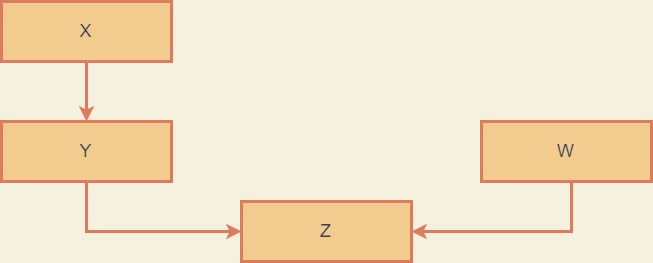Hybrid Inheritance

In this type two types of inheritance are use single inheritance and multilevel inheritance.

Single Inheritance:  W is a base class, Z is a derived class. Single inheritance has only one base class and one derived class.

Multilevel Inheritance:                X is s base class for Y, Y is a derived class from X and base class for Z. Z is a derived class from Y.

Example:   Write a program to show the hybrid inheritance.

or

Write a program to create a derived class from multiple base class.

or

Write a program to create hybrid inheritance and take data members such as name of the player, age, city and game name.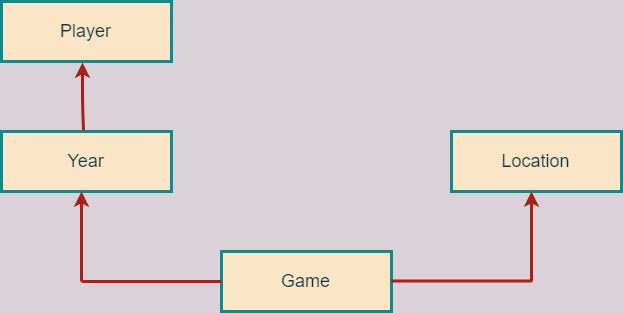Hybrid Inheritance Example

Output

Note: In this type two types of inheritance are used single inheritance and multilevel inheritance.

Single Inheritance:  location is a base class, game is a derived class.

Single level inheritance has only one base class and one derived class.

Class game inherits the properties of class location.

Multilevel Inheritance: player is a base class for Year, Year is a derived class from player .

year is a  base class for game. game is a derived class from Year. Class game inherits the properties of both  class player & year.

Multipath Inheritance : When a class derived from two or more classes that are derived from same base class such type of inheritance is known as multipath inheritance.

W is a base class and X & Y are derived class. The class X & Y are the derived  from W.  Further Z is derived from X & Y.

Means X & Y  is a base class for Z and Z is a derived class. Here, ambiguity is generated.

Hence, virtual keyword is used to avoid ambiguity.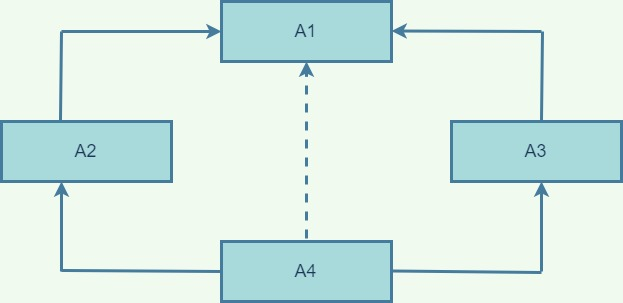Multipath Inheritance Example

Example:

In the above example, A1 is a base class. The class A2 & A3 are the derived  from class A1. Both A2 and A3 can access the variables/data of class A1 .

Further A4 is derived from A2 & A3.

If we try to access variable a1 of class A1 from class A4, Here, ambiguity is generated &  compiler shows error message.

Member is ambiguous : “A1:a1” and “A1:a1”

The derived class A4 has two sets of data member of class A1 through the middle base classes A2 and A3.

The A1 is inherited twice.

Hence, virtual keyword is used to avoid ambiguity.

Categories C++